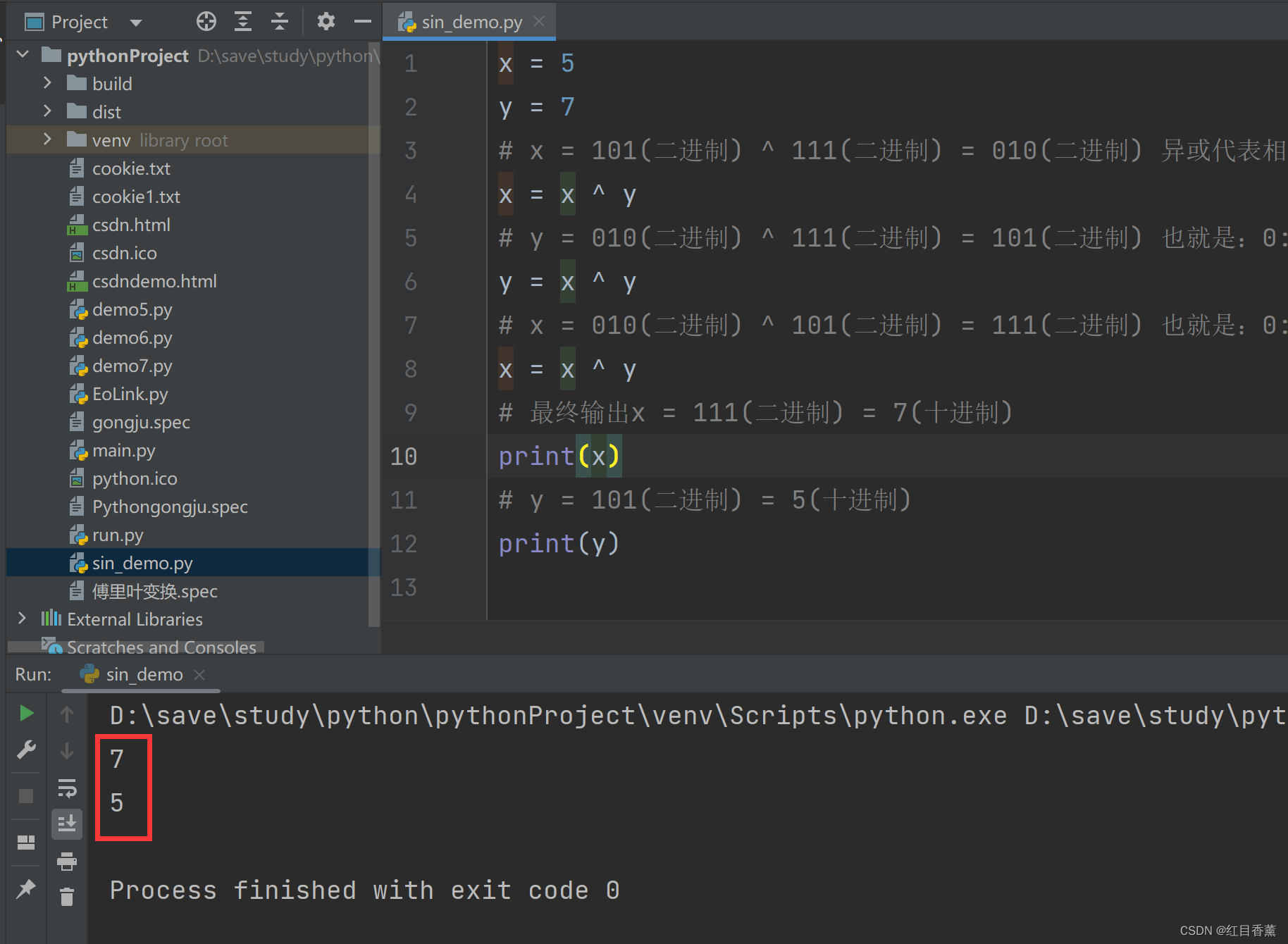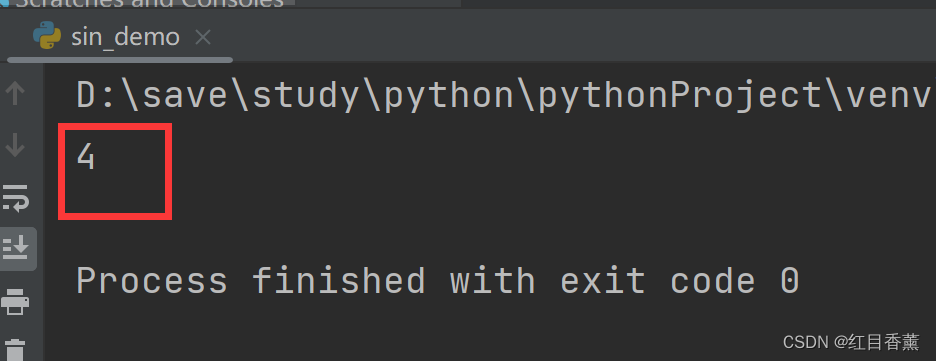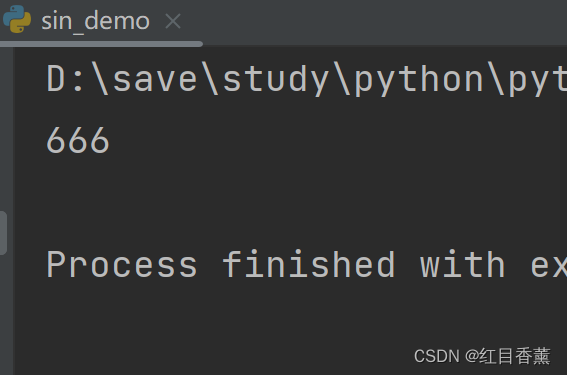> 文档中心 > Python异或运算符示例

# Python异或运算符示例

## 异或

 a b a^b 0假 0假 0假 0假 1真 1真 1真 0假 1真 1真 1真 0假

## 异或的性质

1、a ^ a=0 任何数字和自己异或结果是0
2、a ^ 0=a 任何数字和0异或还是他自己
3、a ^ b = b ^ a 异或运算具有交换律
4、a ^ (b ^ c) = (a ^ c) ^ b 异或运算具有结合律

## 示例1：值交换

x=5 y=7将两个变量的值通过异或运算符进行交换

x = 5
y = 7
# x = 101(二进制) ^ 111(二进制) = 010(二进制) 异或代表相同取反，不同取正 1:1-0:1-1:1 = 010 二进制的010等于10进制的2
x = x ^ y
# y = 010(二进制) ^ 111(二进制) = 101(二进制) 也就是：0:1-1:1-0:1 = 101 二进制101等于10进制的5
y = x ^ y
# x = 010(二进制) ^ 101(二进制) = 111(二进制) 也就是：0:1-1:0-0:1 = 111 二进制的111等于10进制的7
x = x ^ y
# 最终输出x = 111(二进制) = 7(十进制)
print(x)
# y = 101(二进制) = 5(十进制)
print(y)

``x = 5y = 7# x = 101(二进制) ^ 111(二进制) = 010(二进制) 异或代表相同取反，不同取正 1:1-0:1-1:1 = 010 二进制的010等于10进制的2x = x ^ y# y = 010(二进制) ^ 111(二进制) = 101(二进制) 也就是：0:1-1:1-0:1 = 101 二进制101等于10进制的5y = x ^ y# x = 010(二进制) ^ 101(二进制) = 111(二进制) 也就是：0:1-1:0-0:1 = 111 二进制的111等于10进制的7x = x ^ y# 最终输出x = 111(二进制) = 7(十进制)print(x)# y = 101(二进制) = 5(十进制)print(y)``## 示例2：找出现一次的元素

[2,2,1]

1

[4,1,2,1,2]

4

### 示例2代码：

``arr = [4, 1, 2, 1, 2]index = 0for i in arr:    index ^= iprint(index)````arr = [4, 1, 2, 3, 1, 2, 3, 1, 2, 3, 2, 1, 2, 3, 4, 666, 1, 2, 3, 2]index = 0for i in arr:    index ^= iprint(index)``